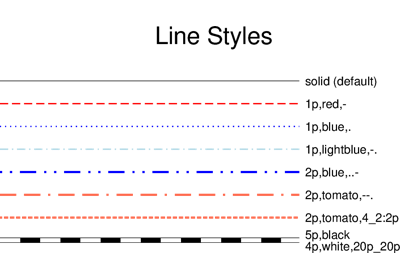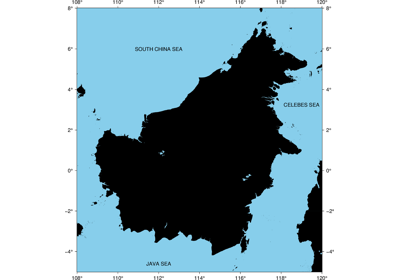# pygmt.Figure.text¶

Figure.text(textfiles=None, x=None, y=None, position=None, text=None, angle=None, font=None, justify=None, **kwargs)

Plot or typeset text strings of variable size, font type, and orientation.

Must provide at least one of the following combinations as input:

• textfiles

• x, y, and text

• position and text

Full option list at https://docs.generic-mapping-tools.org/latest/text.html

Aliases:

• B = frame

• C = clearance

• D = offset

• G = fill

• J = projection

• N = no_clip

• R = region

• V = verbose

• W = pen

• X = xshift

• Y = yshift

• p = perspective

• t = transparency

Parameters
• textfiles (str or list) – A text data file name, or a list of filenames containing 1 or more records with (x, y[, angle, font, justify], text).

• x/y (float or 1d arrays) – The x and y coordinates, or an array of x and y coordinates to plot the text

• position (str) –

Sets reference point on the map for the text by using x,y coordinates extracted from region instead of providing them through x and y. Specify with a two letter (order independent) code, chosen from:

• Horizontal: L(eft), C(entre), R(ight)

• Vertical: T(op), M(iddle), B(ottom)

For example, position=”TL” plots the text at the Upper Left corner of the map.

• text (str or 1d array) – The text string, or an array of strings to plot on the figure

• angle (int, float, str or bool) – Set the angle measured in degrees counter-clockwise from horizontal. E.g. 30 sets the text at 30 degrees. If no angle is explicitly given (i.e. angle=True) then the input textfile(s) must have this as a column.

• font (str or bool) – Set the font specification with format “size,font,color” where size is text size in points, font is the font to use, and color sets the font color. E.g. “12p,Helvetica-Bold,red” selects a 12p red Helvetica-Bold font. If no font info is explicitly given (i.e. font=True), then the input textfile(s) must have this information in one of its columns.

• justify (str or bool) – Set the alignment which refers to the part of the text string that will be mapped onto the (x,y) point. Choose a 2 character combination of L, C, R (for left, center, or right) and T, M, B for top, middle, or bottom. E.g., BL for lower left. If no justification is explicitly given (i.e. justify=True), then the input textfile(s) must have this as a column.

• projection (str) – Required if this is the first plot command. Select map projection.

• region (str or list) – Required if this is the first plot command. 'xmin/xmax/ymin/ymax[+r][+uunit]'. Specify the region of interest.

• clearance (str) – [dx/dy][+to|O|c|C] Adjust the clearance between the text and the surrounding box [15%]. Only used if pen or fill are specified. Append the unit you want (‘c’ for cm, ‘i’ for inch, or ‘p’ for point; if not given we consult ‘PROJ_LENGTH_UNIT’) or ‘%’ for a percentage of the font size. Optionally, use modifier ‘+t’ to set the shape of the textbox when using fill and/or pen. Append lower case ‘o’ to get a straight rectangle [Default]. Append upper case ‘O’ to get a rounded rectangle. In paragraph mode (paragraph) you can also append lower case ‘c’ to get a concave rectangle or append upper case ‘C’ to get a convex rectangle.

• fill (str) – Sets the shade or color used for filling the text box [Default is no fill].

• offset (str) – [j|J]dx[/dy][+v[pen]] Offsets the text from the projected (x,y) point by dx,dy [0/0]. If dy is not specified then it is set equal to dx. Use offset=’j’ to offset the text away from the point instead (i.e., the text justification will determine the direction of the shift). Using offset=’J’ will shorten diagonal offsets at corners by sqrt(2). Optionally, append ‘+v’ which will draw a line from the original point to the shifted point; append a pen to change the attributes for this line.

• pen (str) – Sets the pen used to draw a rectangle around the text string (see clearance) [Default is width = default, color = black, style = solid].

• no_clip (bool) – Do NOT clip text at map boundaries [Default is False, i.e. will clip].

• verbose (str) –

Select verbosity level [Default is w], which modulates the messages written to stderr. Choose among 7 levels of verbosity:

• q - Quiet, not even fatal error messages are produced

• e - Error messages only

• w - Warnings [Default]

• t - Timings (report runtimes for time-intensive algorthms);

• i - Informational messages (same as “verbose=True”)

• c - Compatibility warnings

• d - Debugging messages

• xshift (str) – [a|c|f|r][xshift]. Shift plot origin in x-direction.

• yshift (str) – [a|c|f|r][yshift]. Shift plot origin in y-direction. Full documentation is at https://docs.generic-mapping-tools.org/latest/gmt.html#xy-full.

• perspective (list or str) – '[x|y|z]azim[/elev[/zlevel]][+wlon0/lat0[/z0]][+vx0/y0]'. Select perspective view and set the azimuth and elevation angle of the viewpoint. Default is [180, 90]. Full documentation is at https://docs.generic-mapping-tools.org/latest/gmt.html#perspective-full.

• transparency (float) – Set transparency level, in [0-100] percent range. Default is 0, i.e., opaque. Only visible when PDF or raster format output is selected. Only the PNG format selection adds a transparency layer in the image (for further processing).

## Examples using pygmt.Figure.text¶